# Tutorial¶

The Tutorial section aims to provide working code samples demonstrating how to use the `abess` library to solve real world issues. In the following pages, the `abess` Python package is used for illustration. The counterpart for R package is available at here.

## Generalized Linear Model¶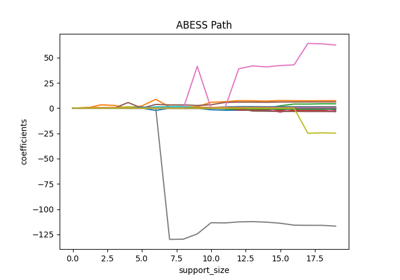Linear Regression

Linear Regression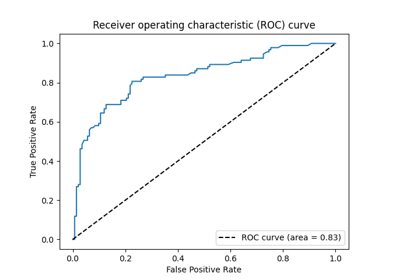Classification: Logistic Regression and Beyond

Classification: Logistic Regression and Beyond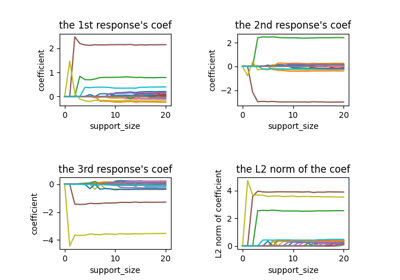Multi-Response Linear Regression

Multi-Response Linear Regression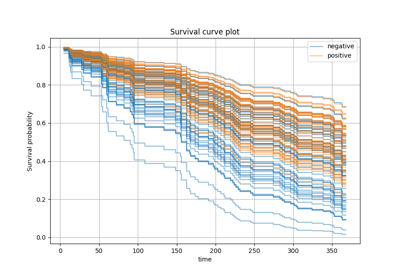Survival Analysis: Cox Regression

Survival Analysis: Cox Regression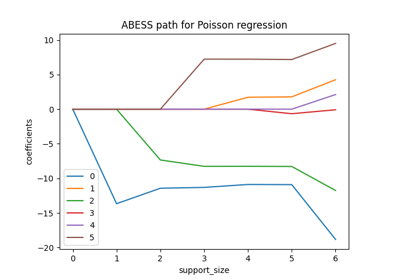Positive Responses: Poisson & Gamma Regressions

Positive Responses: Poisson & Gamma Regressions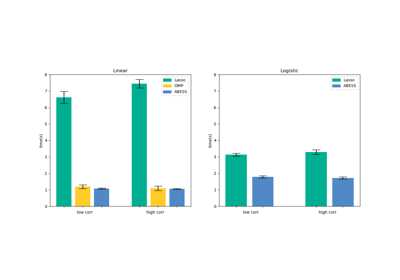Power of abess Library: Empirical Comparison

Power of abess Library: Empirical ComparisonABESS Algorithm: Details

ABESS Algorithm: Details

## Principal Component Analysis¶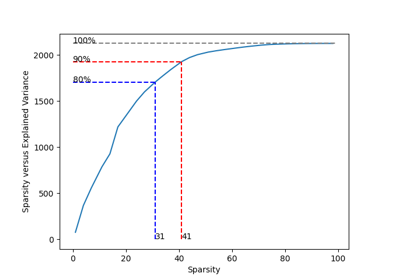Principal Component Analysis

Principal Component Analysis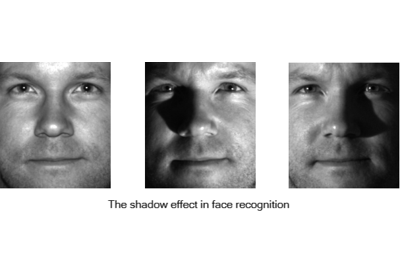Robust Principal Component Analysis

Robust Principal Component Analysis

When analyzing the real world datasets, we may have the following targets:

1. identifying predictors when group structure are provided (a.k.a., best group subset selection);

2. certain variables must be selected when some prior information is given (a.k.a., nuisance regression);

3. selecting the weak signal variables when the prediction performance is mainly interested (a.k.a., regularized best-subset selection).

These targets are frequently encountered in real world data analysis. Actually, in our methods, the targets can be properly handled by simply change some default arguments in the functions. In the following content, we will illustrate the statistic methods to reach these targets in a one-by-one manner, and give quick examples to show how to perform the statistic methods in `LinearRegression` and the same steps can be implemented in all methods.

Besides, `abess` library is very flexible, i.e., users can flexibly control many internal computational components. Specifically, users can specify: (i) the division of samples in cross validation (a.k.a., cross validation division), (ii) specify the initial active set before splicing (a.k.a., initial active set), and so on. We will also describe these in the following.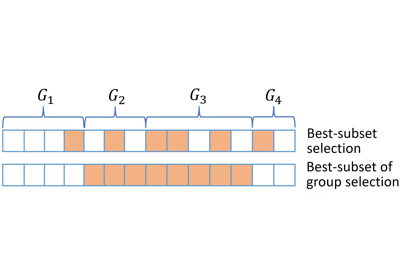Best Subset of Group Selection

Best Subset of Group Selection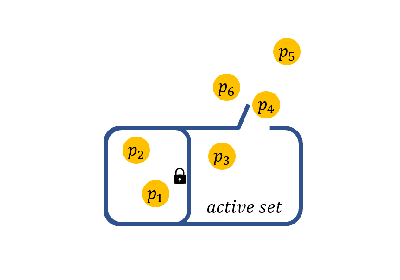Nuisance Regression

Nuisance Regression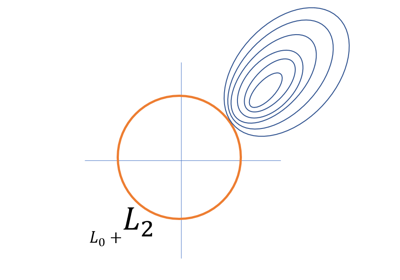Regularized Best Subset Selection

Regularized Best Subset SelectionCross-Validation Division

Cross-Validation DivisionInitial Active Set

Initial Active Set

## Computational Tips¶

The generic splicing technique certifiably guarantees the best subset can be selected in a polynomial time. In practice, the computational efficiency can be improved to handle large scale datasets. The tips for computational improvement are applicable for:

1. ultra-high dimensional data via

• feature screening;

• focus on important variables;

2. large-sample data via

• golden-section searching;

• early-stop scheme;

3. sparse inputs via

• sparse matrix computation;

4. specific models via

• covariance update for `LinearRegression` and `MultiTaskRegression`;

• quasi Newton iteration for `LogisticRegression`, `PoissonRegression`, `CoxRegression`, etc.

More importantly, the technique in these tips can be use simultaneously. For example, `abess` allow algorithms to use both feature screening and golden-section searching such that algorithms can handle datasets with large-sample and ultra-high dimension. The following contents illustrate the above tips.

Besides, `abess` efficiently implements warm-start initialization and parallel computing, which are very useful for fast computing. To help use leverage them, we will also describe their implementation details in the following.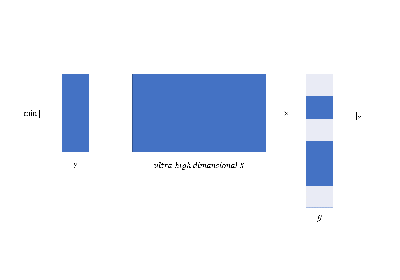Ultra-High dimensional data

Ultra-High dimensional data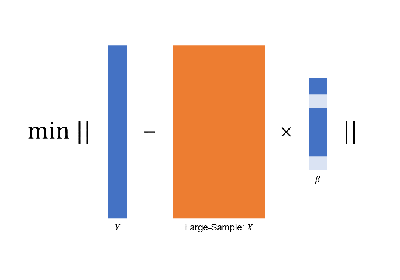Large-Sample Data

Large-Sample Data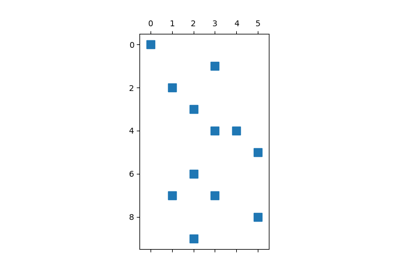Sparse Inputs

Sparse InputsSpecific Models

Specific Models